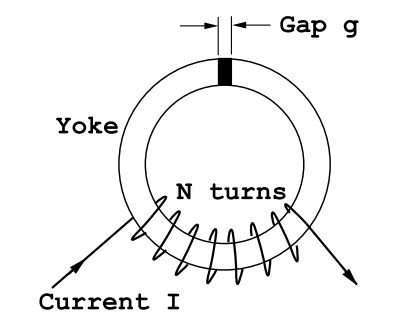$$\require{cancel}$$

# 6.4: Electromagnets

Consider the electromagnet shown in Figure $$\PageIndex{13}$$. As a first approximation let the B-field in the ferromagnetic yoke be uniform with the same value B Teslas everywhere. The field in the gap measured along the centerline will also be B to a good approximation. This follows from the Maxwell equation $$\div B=0$$ which requires the normal component of B to be continuous across a material discontinuity. If the gap field is B then the H-field in the gap is Hg = B/µ0. The permeability of free space, µ0 = 4$$\pi$$ × 10−7 , is a small number therefore Hg will be quite large: if B=1.0 Tesla then Hg = 7.96×105 Amps/m. This H-field is much larger than H within the soft ferromagnetic yoke material. For example, in iron the field H cannot exceed 100 Amps/m if B= 1.0 Tesla, see Figure (6.3). According to another Maxwell equation for the static magnetic field

$\operatorname{curl}(\vec{\text{H}})=\vec{\text{J}} , \nonumber$

or

$\oint_{C} \vec{\text{H}} \cdot \text{d} \vec{\text{L}}=\iint_{A r e a} \vec{\text{J}} \cdot \text{d} \vec{\text{A}} , \label{6.5}$

from Stokes’ theorem where the Area of the surface integration is bounded by the curve C.Figure $$\PageIndex{13}$$: An electromagnet consisting of a soft ferromagnetic yoke wound with N turns of wire and containing a gap of width g meters. The length of the ferromagnetic yoke along its center line is L meters.

Apply Equation (\ref{6.5}) to the closed line running along the centerline of the magnet. The integral of the current density over the area bounded by the magnet center line is just NI so that the line integral of H becomes

$\text{L} \text{H}+\text{g} \text{H}_{\text{g}}=\text{NI} , \nonumber$

where L is the length of the path in the ferromagnetic yoke and g is the width of the gap. Given a value for the B-field one can look up the corresponding value of the H-field from the ferromagnetic hysteresis loop. Then the current required to produce that B-field is

$\text{I}=\frac{1}{\text{N}}\left(\text{LH}+\left[\text{gB} / \mu_{0}\right]\right) . \label{6.6}$

In this way one can construct a graph of B vs. I corresponding to various points on the B-H loop. It should be noted that this simple construction fails when the ferromagnet approaches magnetic saturation.